# Fractions On A Number Line | Good To Know | WSKG

##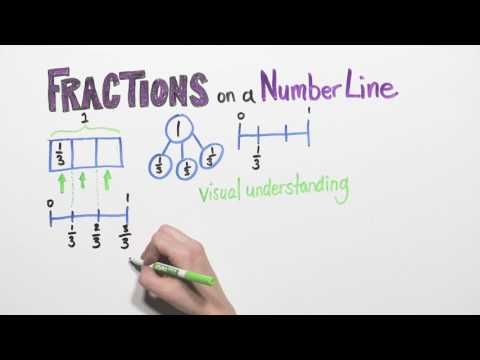By WSKG Public Media

Understand a fraction as a number on the number line; represent fractions on a number line diagram.# Plot Fractions on the Number Line

##By MathwithMrAlmeida

This video plots 6 fractions and operations with fractions on the number line. It ends by plotting all the same fractions on the number line and identify a pair of equivalent fractions and comparing each number's distance from zero.# Fractions and the Number Line - 3rd Grade

##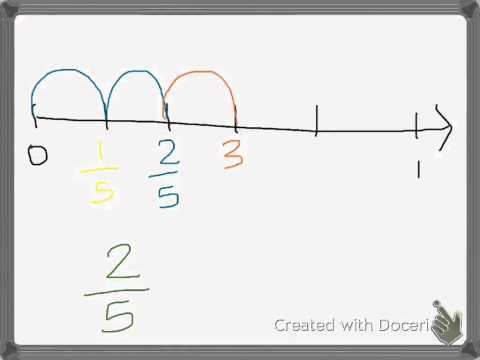By Hellothere1130

represent fractions on the number line# Fractions on a number line | Fractions | 3rd grade | Khan Academy

##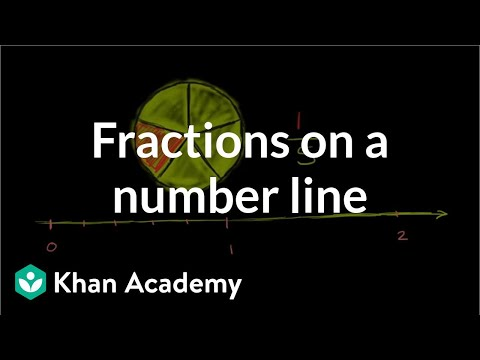By Khan Academy

Graph and locate fractions on a number line.# Grade 3 Math #8.5, Fractions on a Number Line

##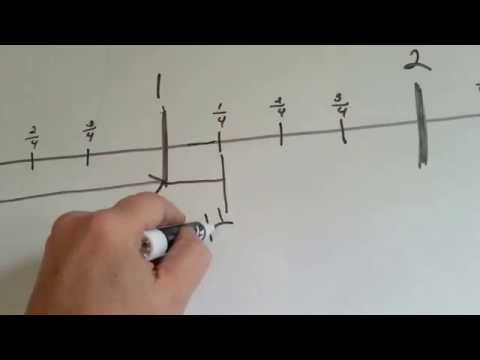By Joann`s School

How fractions are found on a number line. Whole numbers, mixed numbers, fractions on a number line# Comparing fractions on a number line | Fractions | 4th grade | Khan Academy

##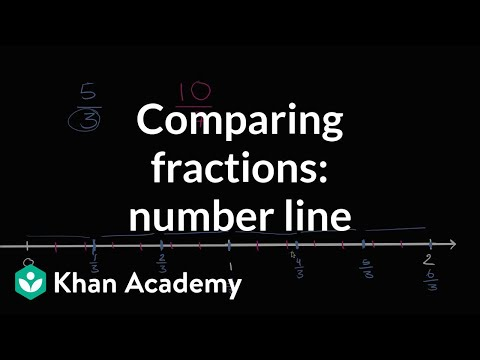By Khan Academy

Comparing fractions on a number line# Fractions on a Number Line - Mr. Pearson Teaches 3rd Grade

##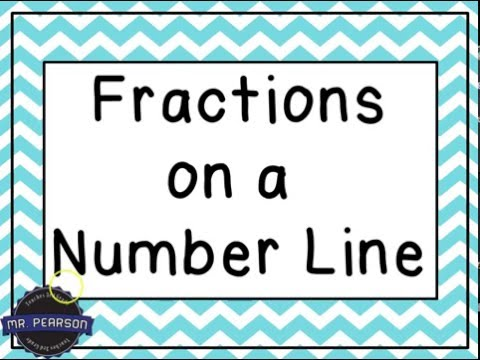By Mr Pearson teaches 3rd grade

This video is designed to teach students how to identify fractions on a number line.# Fractions on the Number Line

##By MathwithMrAlmeida

In this Singapore math lesson Mr. Almeida represents fractions on the number line by first defining how many parts make 1 whole. Then naming each part a fractional unit and counting that many units. This illustrates Common Core math standard 3.NF2.b.# Plotting Fractions on a Number Line

##By mathlablady4

The instructor first reviews how to plot whole numbers on a number line. She then relates the same concept to fractions. In the video lesson she plots both proper and improper fractions. Very clear instruction and easy for students to visualize the concept.# Equivalent fractions using the number line (3.NF.3A)

##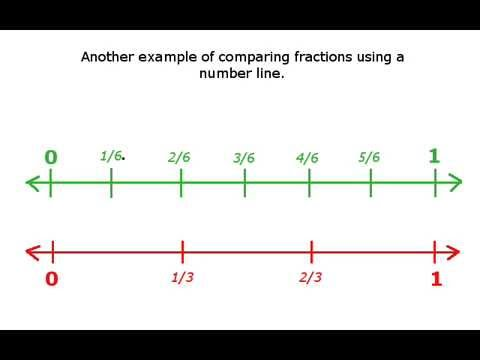By Far East Software

Understand two fractions as equivalent (equal) if they are the same size, or the same point on a number line.# [3.NF.2b-1.5] Fractions on number line - Common Core Standard

##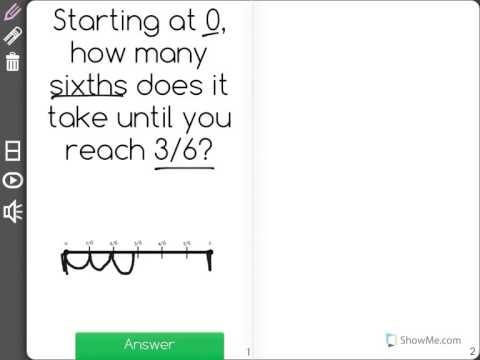By Freckle education

Represent a fraction a/b by marking off lengths 1/b from 0, recognizing that the resulting interval has a size a/b and that its endpoint locates a/b on the number line.# Fractions on the number line

##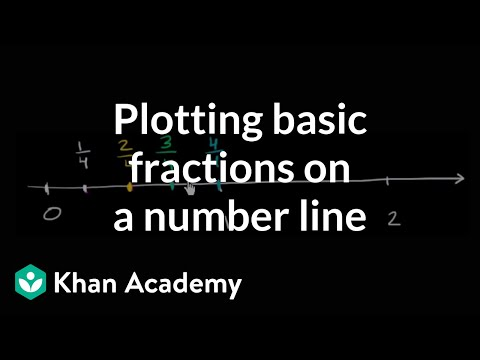By Khan Academy

Fractions on the number line# Fractions on the number line

##By Khan Academy

Fractions on the number line# Fractions on the number line

##By Khan Academy

Graph and locate fractions on a number line.# Fractions on the number line

##By Khan Academy

Graph and locate fractions on a number line.# Equivalent fractions on the number line

##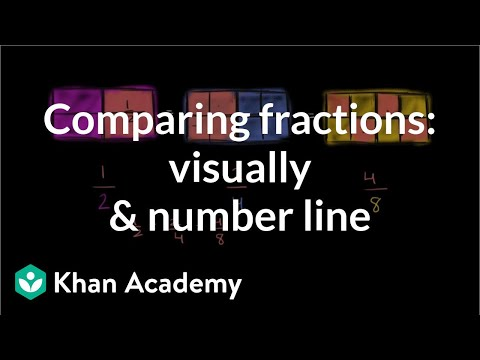By Khan Academy

Compare fractions by graphing them a number line and drawing fraction models.# Equivalent fractions on the number line

##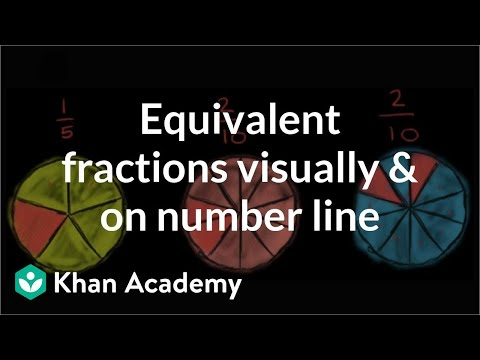By Khan Academy

Use number lines and fraction models to show equivalent fractions.# Equivalent fractions on the number line

##By Khan Academy

Compare fractions by graphing them a number line and drawing fraction models.# Equivalent fractions on the number line

##By Khan Academy

Compare fractions by graphing them a number line and drawing fraction models.# Equivalent fractions on the number line

##By Khan Academy

Use number lines and fraction models to show equivalent fractions.